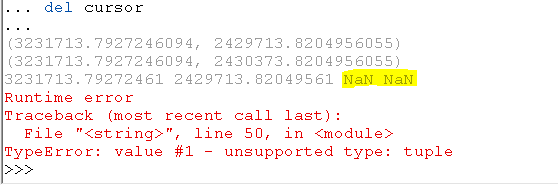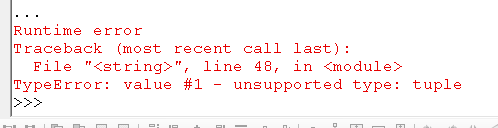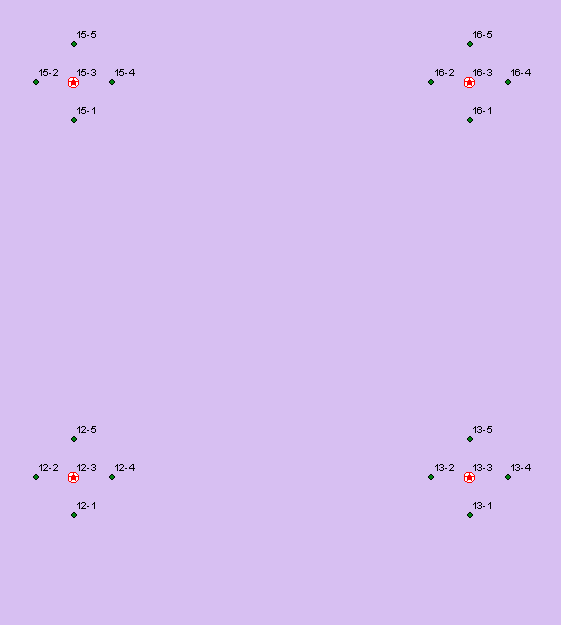# Tuple Error Insert Cursor

1054
7
10-03-2016 12:37 PMRegular Contributor

My brain is fried...if anyone can/wants to modify the title of the post please do so. I'll attempt to post this w/o giving the background of the tool...if that is necessary I'll happily provide it. Here is my code:

``````import arcpy, os, sys, math

#Environment settings
outGDB = arcpy.env.scratchGDB
arcpy.env.workspace = outGDB
arcpy.env.overwriteOutput = True

#Local Variables
#startingPt = arcpy.GetParameterAsText(0)
#sampleNumber = int(arcpy.GetParameterAsText(1))
#interDist = int(arcpy.GetParameterAsText(2))
startingPt = "startingPoints"
interDist = 660
outName = "cruisePoints"

#Create target point FC
sr = arcpy.Describe(startingPt).spatialReference
outPoint = arcpy.CreateFeatureclass_management(outGDB,outName,"POINT","","","",sr)
arcpy.AddField_management(outPoint, "CLUSTER_ID", "TEXT", "", "", "", "", "NULLABLE", "NON_REQUIRED", "")
arcpy.AddField_management(outPoint, "PLOT_ID", "TEXT", "", "", "", "", "NULLABLE", "NON_REQUIRED", "")

#Open Insert Cursor
insert = arcpy.da.InsertCursor(outPoint,["SHAPE@XY","CLUSTER_ID","PLOT_ID"])

fields = ["SHAPE@X", "SHAPE@Y", "OID@"]
with arcpy.da.SearchCursor(startingPt, fields) as cursor:

for row in cursor:
#Set Start points
startX = row
startY = row
cluster = row
print(startX,startY)
northX = row
northY = row + interDist
print(northX, northY)
eastX = row + interDist
eastY = row
southX = row
southY = row - interDist
westX = row - interDist
westY = startY
start = arcpy.Point(startX, startY)
print(start)
north = arcpy.Point(northX, northY)
east = arcpy.Point(eastX, eastY)
south = arcpy.Point(southX, southY)
west = arcpy.Point(westX, westY)
insert.insertRow([start,row,cluster])
insert.insertRow([north,row,cluster])
insert.insertRow([east,row,cluster])
insert.insertRow([south,row,cluster])
insert.insertRow([west,row,cluster])

del cursor‍‍‍‍‍‍‍‍‍‍‍‍‍‍‍‍‍‍‍‍‍‍‍‍‍‍‍‍‍‍‍‍‍‍‍‍‍‍‍‍‍‍‍‍‍‍‍‍‍‍‍‍‍‍‍‍‍``````

When pasting into the Python Console in Desktop I get the following error (note the third print as well)1 Solution

Accepted SolutionsbyMVP Esteemed Contributor

What if you try

``insert.insertRow([(startX, startY),row,cluster])‍‍‍``

``insert.insertRow([start,row,cluster])‍‍‍``

and do similar with the other entries.

You created the insert cursor using SHAPE@XY, but you are trying to pass an arcpy.Point object instead of a tuple representing an X, Y.

7 RepliesbyMVP Esteemed Contributor

What if you try

``insert.insertRow([(startX, startY),row,cluster])‍‍‍``

``insert.insertRow([start,row,cluster])‍‍‍``

and do similar with the other entries.

You created the insert cursor using SHAPE@XY, but you are trying to pass an arcpy.Point object instead of a tuple representing an X, Y.Regular Contributor

bixb0012 Thanks for the response. I tried the modification (and I see your point now...no pun intended), but I'm still getting the same error:Below is a portion of the code, line 48 from the error is line 27 below.

``````#Open Insert Cursor
insert = arcpy.da.InsertCursor(outPoint,["SHAPE@XY","CLUSTER_ID","PLOT_ID"])

fields = ["SHAPE@X", "SHAPE@Y", "OID@"]
with arcpy.da.SearchCursor(startingPt, fields) as cursor:

for row in cursor:
#Set Start points
startX = row
startY = row
cluster = row
northX = row
northY = row + interDist
eastX = row + interDist
eastY = row
southX = row
southY = row - interDist
westX = row - interDist
westY = row
#start = arcpy.Point(startX, startY)
#print(start)
#north = arcpy.Point(northX, northY)
#east = arcpy.Point(eastX, eastY)
#south = arcpy.Point(southX, southY)
#west = arcpy.Point(westX, westY)
insert.insertRow([(startX,startY),row,cluster])
insert.insertRow([(northX,northY),row,cluster])
insert.insertRow([(eastX,eastY),row,cluster])
insert.insertRow([(southX,southY),row,cluster])
insert.insertRow([(westX,westY),row,cluster])‍‍‍‍‍‍‍‍‍‍‍‍‍‍‍‍‍‍‍‍‍‍‍‍‍‍‍‍‍‍‍``````

Dan_Patterson‌ I'm glad I finally found your codeblock formatting write-up! Thanks for that...it was driving me nuts posting stuff as a quote before.byMVP Frequent Contributor

I am not sure that this is going to work :

``````outPoint = arcpy.CreateFeatureclass_management(outGDB,outName,"POINT","","","",sr)
arcpy.AddField_management(outPoint, "CLUSTER_ID", "TEXT", "", "", "", "", "NULLABLE", "NON_REQUIRED", "")
arcpy.AddField_management(outPoint, "PLOT_ID", "TEXT", "", "", "", "", "NULLABLE", "NON_REQUIRED", "")

#Open Insert Cursor
insert = arcpy.da.InsertCursor(outPoint,["SHAPE@XY","CLUSTER_ID","PLOT_ID"])``````

The result of the CreateFeatureclass will be a result object, not necessarily a pointer to the table on disk.

Rather set outPoint explicitly.

Like

outPoint = os.path.join(outGDB, outName)byMVP Frequent Contributor

But, having looked at that again, I think Joshua is right.

Why are you pushing the value of row which is a tuple of the entire data row, into the attribute CLUSTER_ID?Regular Contributor

Ok, so now we get to the background. I have another script which creates points spaced at a regular interval. These are the 'starting points' for field sampling. The field tech navigates to that location, does the sampling work, and then has 4 other points associated with the particular starting point which they need to conduct the same work at. A total of 5 points. These five points constitute a 'cluster'. The points in each each cluster need to be identified/associated with the cluster ("CLUSTER_ID"), but also have a unique value ("PLOT_ID"); concatenating the two would give you a unique value for each point for the entire project.

FWIW, the error raised has to do with 'value 1' which seems to be the XY.

Anyway, here's a graphic of what I'm trying to achieve.Regular Contributor

I understand what you guys are getting at now regarding the 'row' (doh!). I've modified the inserts, and the script now runs correctly!

Can I get some guidance as to who to mark as the correct answer here? Everyone helped in one way or another. Should I modify the title of the post at all to better reflect what was going on here?

Thanks so much!

``````import arcpy, os, sys, math
#Environment settings
outGDB = arcpy.env.scratchGDB
arcpy.env.workspace = outGDB
arcpy.env.overwriteOutput = True

#Local Variables
#startingPt = arcpy.GetParameterAsText(0)
#sampleNumber = int(arcpy.GetParameterAsText(1))
#interDist = int(arcpy.GetParameterAsText(2))
startingPt = "startingPoints"
interDist = 660
outName = "cruisePoints"

#Create target point FC
sr = arcpy.Describe("frontier").spatialReference
outPoint = arcpy.CreateFeatureclass_management(outGDB,outName,"POINT","","","",sr)
arcpy.AddField_management(outPoint, "CLUSTER_ID", "TEXT", "", "", "", "", "NULLABLE", "NON_REQUIRED", "")
arcpy.AddField_management(outPoint, "PLOT_ID", "TEXT", "", "", "", "", "NULLABLE", "NON_REQUIRED", "")

#Open Insert Cursor
insert = arcpy.da.InsertCursor(outPoint,["SHAPE@XY","CLUSTER_ID","PLOT_ID"])

fields = ["SHAPE@X", "SHAPE@Y", "OID@"]
with arcpy.da.SearchCursor(startingPt, fields) as cursor:

for row in cursor:
#Set Start points
startX = row
startY = row
cluster = row
northX = row
northY = row + interDist
eastX = row + interDist
eastY = row
southX = row
southY = row - interDist
westX = row - interDist
westY = row
insert.insertRow([(startX, startY),cluster,"1"])
insert.insertRow([(northX, northY),cluster,"2"])
insert.insertRow([(eastX, eastY),cluster,"3"])
insert.insertRow([(southX, southY),cluster,"4"])
insert.insertRow([(westX, westY),cluster, "5"])

del cursor‍‍‍‍‍‍‍‍‍‍‍‍‍‍‍‍‍‍‍‍‍‍‍‍‍‍‍‍‍‍‍‍‍‍‍‍‍‍‍‍‍‍‍‍‍‍‍‍‍‍‍‍‍‍‍‍‍‍‍‍‍‍‍‍‍‍‍‍‍‍‍‍‍‍‍‍‍‍‍‍‍‍‍‍‍‍‍‍‍‍‍‍‍‍‍‍``````byMVP Esteemed Contributor

Regarding giving credit and marking correct answers, it isn't always straightforward.  I think most people would say mark the response that was most helpful (judgment call, obviously) correct and mark the other ones helpful.  If it is too difficult to pick one over the others, think about marking the first equally helpful one as correct.  If even that doesn't feel right, mark all of the helpful ones as helpfuls and the question as "assumed answered."